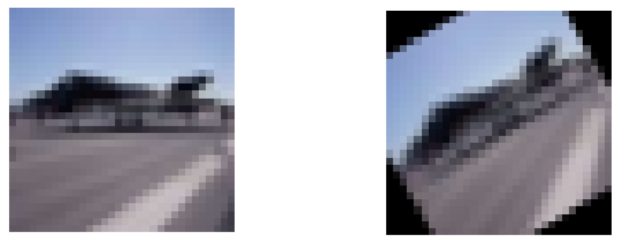# Random transforms and targets

A bit more on the data augmentation process which has been the biggest challenge of this rewriting of the fastai library. I’ve already explained here how we think the new pipeline will be more efficient (still waiting for the pytorch team to optimize two functions to compare with the other existing libraries). We then have worked a lot on insuring that it works well with rectangular images, to be able to do training with rectangular images.

The next challenge is to introduce randomness to them, especially when the target needs to be modified the way the original image is. When we just want to classify images (like dogs vs cats) this isn’t a problem, but in other tasks such as segmentation, object detection with bounding box, pose detection… the target is linked to the image and needs to change if you do a small rotation/zoom/crop…

## Randomness

In terms of API, we’re still polishing and refactoring so it’s not completely precise. The big lines is that each transform is defined as a function with a decorator. For instance this is the flip transform:

``````@reg_transform
def flip_lr(x) -> TfmPixel: return x.flip(2)
``````

The return type is very important since it’ll tell the fastai library the type of the transform (so that they are applied in the right order in the end). Depending on the type, the function can also be a bit different. For instance an affine transform like a rotation is defined by its matrix:

``````@reg_transform
def rotate(degrees:uniform) -> TfmAffine:
angle = degrees * math.pi / 180
return [[cos(angle), -sin(angle), 0.],
[sin(angle),  cos(angle), 0.],
[0.        ,  0.        , 1.]]
``````

then the library will apply all the affine transforms at once as explained here.

The important part, is that the transforms are defined in a deterministic manner (flip takes a tensor and flips it, rotate defines a matrix that depends of the degrees argument) and the API will create a new function with the suffix _tfm from it: flip_tfm or rotate_tfm for instance. The difference is those are now some kinds of meta-functions, and instead of passing them directly a tensor, we will define how we want the random arguments to behave.

``````my_tfm = random_tfm(degrees=(-30,30))
``````

Here my_tfm will be a random rotation with degrees picked (uniformly) between -30 and 30. All _tfm automatically get a new argument p that represents the probability of it being applied (default 1, always applied).

``````my_tfms = [random_tfm(degrees=(-30,30), p=0.25), flip_lr_tfm(p=0.5)]
``````

Here my_tfms combines a random rotation like before, but only applied with probability 0.25, and a random horizontal flip with probability 0.5.

The point is to make it as easy as possible for contributors to fastai to write new transforms, the API taking care of all the randomness and dealing with doing the same thing to the image and the target (if needed). This will look like something:

``````tfms.resolve()
x = tfms.apply(x)
if tfm_y != TfmY.No: y = tfms.apply(y, tfm_y)
``````

In the first line, the API picks the random numbers that will determine each one of the transforms (the degree and the p in the previous examples), then we apply them to our x (an image probably) and to our y if the tfm_y class (that represents the type of problem we have) is set to something different than: don’t touch y.

## Target transforms type

Depending on the type of problem we have, we can separate the TfmY types into five categories (I believe).

TfmY.No: The regular classification problem, don’t touch the target.This is an airplane and… still a airplane.

TfmY.Pixel: Types of problems where the target is an image, like the enhance notebook. In this case, we want all the transforms applied to the target (here input is up, target is below).

TfmY.Mask: Types of problems where the target is a segmentation mask, like the carvana notebook. In this case, we want all the transforms applied to the target except the ones that have to do with lightning/contrast. Also we have 0/1 values for the pixel at the beginning and want to keep that at the end (for multiple classes, have a target with multiple channels).

TfmY.Coord: Types of problem where the targets are a set of points (like the human poses). In this case, not only do we want the transforms that affects the coordinates to be applied to the target, but since it’s not a regular image, we have to be extra careful.

TfmY.Bbox: Types of problem where the targets is a bounding box. It’s a bit like the coords TfmY but this time we have to adapt the final result to get a nice bounding box that takes all the object.

Hopefully we’ll have all of this nicely rounded up in the next few weeks into a simple API, and I’ll be able to show how to obtain all those pictures very easily with fastai_v1!

12 Likes

I just pushed a fairly significant update to the transforms API, such that much of this info is now out of date - will endeavor to update it sometime soon-ish.

4 Likes